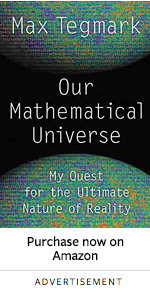# How to see yourself in a world where only math is realMax Tegmark at Nautilus:

The idea of spacetime does more than teach us to rethink the meaning of past and future. It also introduces us to the idea of a mathematical universe. Spacetime is a purely mathematical structure in the sense that it has no properties at all except mathematical properties, for example the number four, its number of dimensions. In my book Our Mathematical Universe, I argue that not only spacetime, but indeed our entire external physical reality, is a mathematical structure, which is by definition an abstract, immutable entity existing outside of space and time.

What does this actually mean? It means, for one thing, a universe that can be beautifully described by mathematics. That this is true for our universe has become increasingly clear over the centuries, with evidence piling up ever more rapidly. The latest triumph in this area is the discovery of the Higgs boson, which, just like the planet Neptune and the radio wave, was first predicted with a pencil, using mathematical equations.

That our universe is approximately described by mathematics means that some but not all of its properties are mathematical. That it is mathematical means that all of its properties are mathematical; that it has no properties at all except mathematical ones. If I’m right and this is true, then it’s good news for physics, because all properties of our universe can in principle be understood if we are intelligent and creative enough. It also implies that our reality is vastly larger than we thought, containing a diverse collection of universes obeying all mathematically possible laws of physics.

more here.